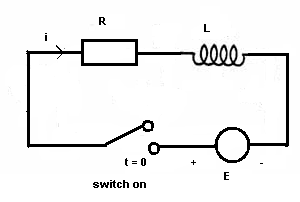# Applications of Differential Equations

We present examples where differential equations are widely applied to model natural phenomena, engineering systems and many other situations.

## Application 1 : Exponential Growth - Population

Let P(t) be a quantity that increases with time t and the rate of increase is proportional to the same quantity P as follows
d P / d t = k P

where d p / d t is the first derivative of P, k > 0 and t is the time.
The solution to the above first order differential equation is given by
P(t) = A ek t

where A is a constant not equal to 0.
If P = P
0 at t = 0, then
P
0 = A e0
which gives A = P
0
The final form of the solution is given by
P(t) = P0 ek t

Assuming P
0 is positive and since k is positive, P(t) is an increasing exponential. d P / d t = k P is also called an exponential growth model.

## Application 2 : Exponential Decay - Radioactive Material

Let M(t) be the amount of a product that decreases with time t and the rate of decrease is proportional to the amount M as follows
d M / d t = - k M

where d M / d t is the first derivative of M, k > 0 and t is the time.
Solve the above first order differential equation to obtain
M(t) = A e- k t

where A is non zero constant.
It we assume that M = M
0 at t = 0, then
M
0 = A e0
which gives A = M
0
The solution may be written as follows
M(t) = M0 e- k t

Assuming M
0 is positive and since k is positive, M(t) is an decreasing exponential. d M / d t = - k M is also called an exponential decay model.

## Application 3 : Falling Object

An object is dropped from a height at time t = 0. If h(t) is the height of the object at time t, a(t) the acceleration and v(t) the velocity. The relationships between a, v and h are as follows:
a(t) = dv / dt , v(t) = dh / dt.
For a falling object, a(t) is constant and is equal to g = -9.8 m/s.
Combining the above differential equations, we can easily deduce the follwoing equation
d
2h / dt 2 = g
Integrate both sides of the above equation to obtain
dh / dt = g t + v
0
Integrate one more time to obtain
h(t) = (1/2) g t
2 + v0 t + h0
The above equation describes the height of a falling object, from an initial height h
0 at an initial velocity v0, as a function of time.

## Application 4 : Newton's Law of Cooling

It is a model that describes, mathematically, the change in temperature of an object in a given environment. The law states that the rate of change (in time) of the temperature is proportional to the difference between the temperature T of the object and the temperature Te of the environment surrounding the object.
d T / d t = - k (T - Te)

Let x = T - Te so that dx / dt = dT / dt
Using the above change of variable, the above differential equation becomes
d x / d t = - k x

The solution to the above differential equation is given by
x = A e
- k t
substitute x by T - Te
T - Te = A e
- k t
Assume that at t = 0 the temperature T = To
To - Te = A e
0
which gives A = To - Te
The final expression for T(t) i given by
T(t) = Te + (To - Te)e
- k t
This last expression shows how the temperature T of the object changes with time.

## Application 5 : RL circuitLet us consider the RL (resistor R and inductor L) circuit shown above. At t = 0 the switch is closed and current passes through the circuit. Electricity laws state that the voltage across a resistor of resistance R is equal to R i and the voltage across an inductor L is given by L di/dt (i is the current). Another law gives an equation relating all voltages in the above circuit as follows:
L di/dt + Ri = E , where E is a constant voltage.
Let us solve the above differential equation which may be written as follows
L [ di / dt ] / [E - R i] = 1
which may be written as
- (L / R) [ - R d i ] / [E - Ri] = dt
Integrate both sides
- (L / R) ln(E - R i) = t + c , c constant of integration.
Find constant c by setting i = 0 at t = 0 (when switch is closed) which gives
c = (-L / R) ln(E)
Substitute c in the solution
- (L / R) ln(E - R i) = t + (-L/R) ln (E)
which may be written
(L/R) ln (E)- (L / R) ln(E - R i) = t
ln[E/(E - Ri)] = t(R/L)
Change into exponential form
[E/(E - Ri)] = e
t(R/L)
Solve for i to obtain
i = (E/R) (1-e
-Rt/L)
The starting model for the circuit is a differential equation which when solved, gives an expression of the current in the circuit as a function of time.

More references on Differential Equations